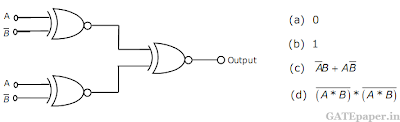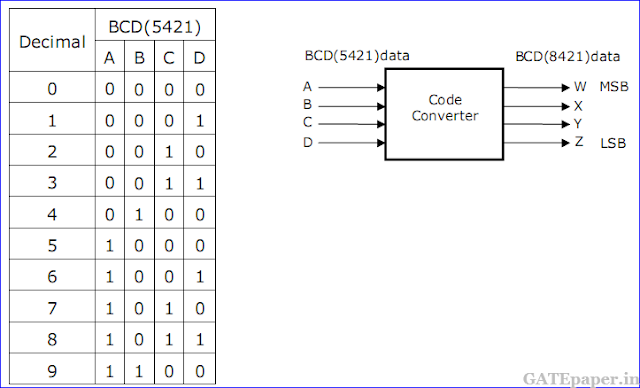### GATE 1995 ECE Video Solutions on Digital Circuits (Digital Electronics)

1. The output of the circuit shown in figure is equal to2. The minimum number of NAND gates required to implement the Boolean function A + AB’ + AB’C is equal to
a. Zero
b. 1
c. 4
d. 7

3. A switch-tail ring counter is made by using a single D flip flop. The resulting circuit is a
a. SR flip flop
b. JK flip flop
c. D flip flop
d. T flip flop

4. When a CPU is interrupted, it
a. Stops executing of instructions
b. Acknowledges interrupt and branches of subroutine
c. Acknowledges interrupt and continues
d. Acknowledges interrupt and waits for the next instruction from the interrupt device
Solution:

5. The minimum number of MOS transistors required to make a dynamic RAM cell is
a. 1
b. 2
c. 3
d. 4

6. An SR latch is a
a. Combinational circuit
b. Synchronous sequential circuit
c. One bit memory element
d. One clock delay element

7. A ‘DMA’ transfer implies
a. Direct transfer of data between memory and accumulator
b. Direct transfer of data between memory and I/O devices without use of µP
c. Transfer of data exclusively within µP registers
d. A fast transfer of data between µP and I/O devices
Solution:

8. An ‘Assembler’ of a microprocessor is used for
a. Assembly of processors in a production line
b. Creation of new program’s using different modules
c. Translation of a program form assembly language to machine language
d. Translation of a higher level language into English text
Solution:

9. For a TTL gate, match the following10. For an ADC, match the following11. A ROM is used to implement the Boolean functions given below.a. What is the minimum size of the ROM required?
b. Determine the data in each location of the ROM

12. A hypothetical CPU has a parallel address bus, a parallel data bus, a RD and WR active LOW signals. Two ROMs of size 4K words each and two RAMs of sizes 16K and 8K words, respectively, are to be connected to the CPU. The memories are to be so connected that they fill the address space of the CU as per the memory map shown in the figure. Assuming tat chip select signals are active LOW.a. What is the number of lines in the address bus of the CPU?
b. Determine the values of address X, Y, Z and W as decimal numbers.
c. Using a 2 to 4 decoder and some additional gates, draw a circuit for the decoding logic.

13. A ‘code converter’ is to be designed to convert from the BCD (5421) code to normal BCD (8421) code. The input BCD combinations for each digit are given below. A block diagram of the converter is shown in figure.
a. Draw K- map for outputs W, X, Y and Z
b. Obtain minimized expression for the outputs W, X, Y and Z